## Oscilloscope Based Spectrum Analysis: Fit Peaks to Enhance Frequency Measurements

### Jon Meek - November 2016

The time domain data for the spectrum used in this example were acquired on a Keysight DSO-X 3024T oscilloscope. Five 500,000 point wave forms were acquired and processed in R (Hanning apodization before FFT and power spectrum output). The resulting 250,001 point frequency domain spectra were added for signal averaging and the result saved for the post-processing exercise shown here. File columns are labeled F (frequency in MHz) and Pwr (power in arbitrary units).

An antenna (a Fanfare FM antenna tuned for the low end of the FM range, but a random length wire produces similar results at the high frequencies examined) was connected to channel 1 of the scope with 50 ohm input impedance. The time base was set to 20 us / division and the volts per division was adjusted to approximately fill the screen.

Note that the analog bandwidth of the DSO-X 3024T is only 200 MHz, but there was enough signal in the 500 MHz region to easily see several peaks with the 5 GSa/s sample rate.

To provide signals with known frequencies a pair of PTS-500 frequency synthesizers were running nearby with short wires on their output connectors and their output power near the possible minimum. The master PTS-500 has an OXCO and is settable to 0.2 Hz, the slave unit uses the clock from the master and is settable to only 0.1 MHz steps. The frequency synthesizers were set to 499.985876 and 490.9 MHz.

This page was produced using R, RMarkdown, and knitr configured to show all code and results.

``````RCSid <- '\$Id: peak_fit_500MHz.Rmd,v 1.4 2016/11/28 23:22:15 meekj Exp \$'

## Sample data fd-5avg-dualfs-2.dat
## Peaks from PTS-500s: 490.9 MHz  & 499.985876  MHz

## Frequency domain spectrum from previously acquired and processed oscilloscope 'RF noise'
File <- '~/lab/R/data/fd-5avg-dualfs-2.dat'

library(wmtsa)                   # Peak detection, uses MASS which overloads dplyr::select
library(ggplot2)                 # Plotting
suppressMessages(library(dplyr)) # Data handling, messages about masked objects are suppresed for presentation cleanliness

``````
``````## Parsed with column specification:
## cols(
##   Chan = col_integer(),
##   F = col_double(),
##   Pwr = col_double()
## )
``````
``````## Setup graphics environment

FigureWidth  <- 16
FigureHeight <- 8

## A custom ggplot2 theme that is decent for HTML reports
theme_jm2 <- theme_bw() +
theme(
plot.title  = element_text(size = rel(1.5), family = 'Helvetica', face = 'bold'),
axis.title  = element_text(size = rel(1.5), colour = "black", face = 'bold'),
axis.text.x = element_text(size = rel(1.5), lineheight = 0.9, colour = "black", vjust = 1, face = 'bold'),
axis.text.y = element_text(size = rel(1.5), lineheight = 0.9, colour = "black", hjust = 1, face = 'bold'),
legend.text = element_text(size = rel(1.3)),
plot.title = element_text(lineheight=.8, face="bold", size = 10),
strip.text.y = element_text(size = rel(1.7), colour = "black", face = 'bold') # Enhance readability of facet labels
)

## A wavlet method to detect peaks using wmtsa package
##
findPeaksCWT <- function(df, SNRmin = 5) {

W <- wavCWT(df\$Pwr)
z <- wavCWTTree(W)
pk <- wavCWTPeaks(z, snr.min = SNRmin, length.min = 2, noise.fun = 'sd', noise.span = 2)

f0 <- display_data\$F # Channel to frequency conversion
df <- display_data\$F - display_data\$F

data.frame(list(F = f0 + df * pk\$x, Height = pk\$y, Chan = pk\$x)) # Return peaks in a data frame
}
``````

## Full Spectrum

``````ggplot(pwr_spectrum) + geom_line(aes(x = F, y = Pwr), color = 'blue') + xlab('F, MHz') + ylab('Power') + ggtitle('Full Spectrum') + theme_jm2
``````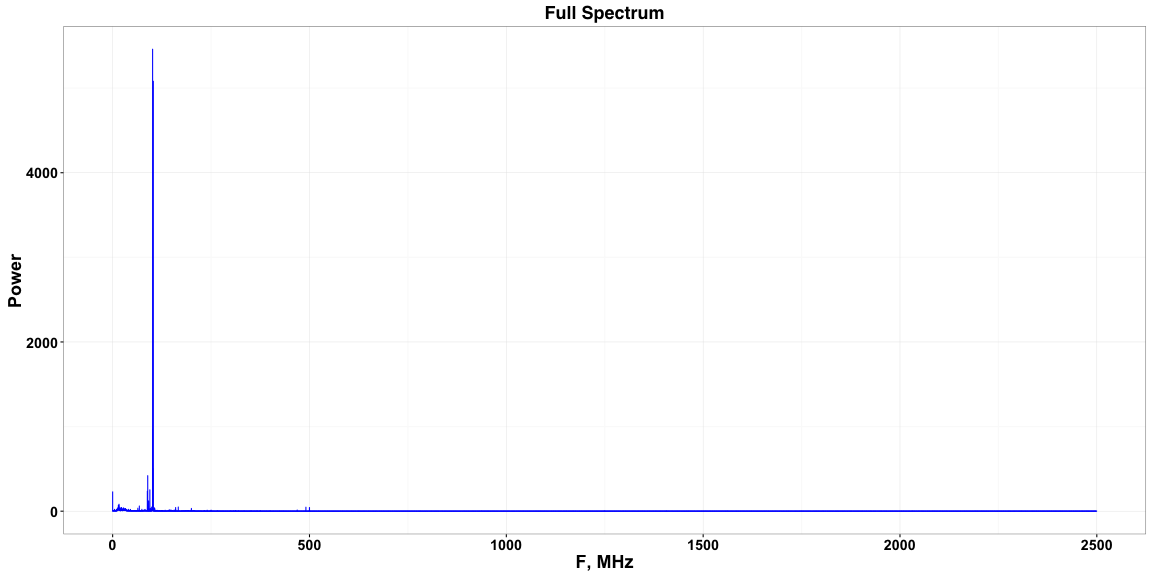## Select Spectrum Segment & Detect Peaks

``````## Select region of interest and detect peaks

DisplayStart <- 465 # MHz
DisplayEnd   <- 505

display_data <- pwr_spectrum %>% filter(F >= DisplayStart & F <= DisplayEnd)

Peaks <- findPeaksCWT(display_data, SNRmin = 10)
Peaks <- Peaks %>% filter(Height > 5)
Peaks                                   # List the detected peaks
``````
``````##        F   Height Chan
## 1 468.76 13.75712  375
## 2 490.91 47.12741 2590
## 3 500.00 45.39824 3499
``````
``````HeightThreshold <- 5
Peaks <- Peaks %>% filter(Height > HeightThreshold)
Peaks # List the peaks
``````
``````##        F   Height Chan
## 1 468.76 13.75712  375
## 2 490.91 47.12741 2590
## 3 500.00 45.39824 3499
``````
``````Title <- 'Frequency Range of Interest'

label_offset <- 0.05 * max(display_data\$Pwr)

ggplot(display_data) + geom_line(aes(x = F, y = Pwr), color = 'blue') +
xlab('F, MHz') + ylab('Power') + ggtitle(Title) +
geom_text(data = Peaks, aes(x = F, y = Height +
label_offset, label = sprintf('%0.1f', F)), size = 7) + theme_jm2
``````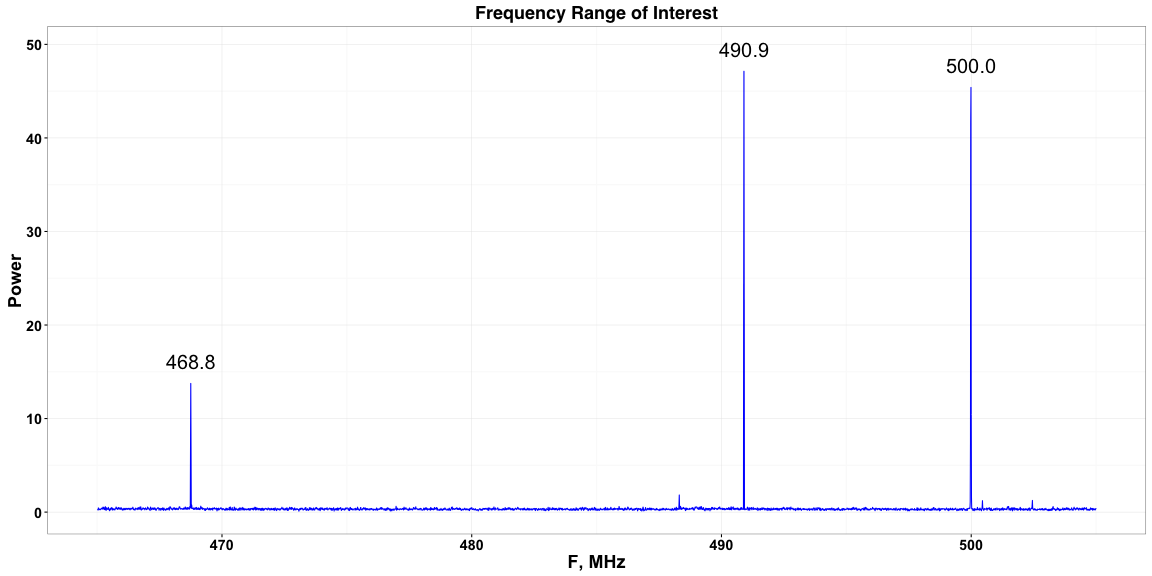The lowest frequency labeled peak above is an unknown signal. The other two labeled peaks are from the frequency synthesizers.

## Fit & Plot the Detected Peaks

``````SigmaToFWHM <- 2.3548200 # Constant to calculate Full Width at Half Maximum

FitChannels <- 10  # Number of points to include in fit
FitPeaks <- NULL   # Data frame with fit peaks data

for (i in 1:nrow(Peaks)) { # Fit Gaussian shape to each detected peak

fit_start_index <- Peaks\$Chan[i] - FitChannels / 2
fit_end_index   <- Peaks\$Chan[i] + FitChannels / 2

data_to_fit <- display_data[fit_start_index:fit_end_index, ]

initial.height <- max(data_to_fit\$Pwr)                       # Initial height is maximum Pwr
initial.mu     <- data_to_fit\$F[which.max(data_to_fit\$Pwr)]  # Initial centroid is channel with maximum Pwr

dF <- data_to_fit\$F - data_to_fit\$F                    # Channel spacing in MHz, use as initial sigma

## Use native R non-linear least squares
res <- nls( Pwr ~ height * exp( -0.5 * ((F - mu) / sigma)^2 ),
start=c(mu=initial.mu, sigma=dF, height=initial.height),
data = data_to_fit)

## Extract the fit results
t.df <- data.frame(list(Centroid   = summary(res)\$parameters["mu", "Estimate"],
CentroidSE = summary(res)\$parameters["mu", "Std. Error"],
Height     = summary(res)\$parameters["height", "Estimate"],
HeightSE   = summary(res)\$parameters["height", "Std. Error"],
Sigma      = summary(res)\$parameters["sigma", "Estimate"],
SigmaSE    = summary(res)\$parameters["sigma", "Std. Error"],
FWHM       = SigmaToFWHM * summary(res)\$parameters["sigma", "Estimate"],
FWHMSE     = SigmaToFWHM * summary(res)\$parameters["sigma", "Std. Error"],
InitialCentroid = initial.mu
))

FitPeaks <- rbind(FitPeaks, t.df) # Build data frame
}

FitPeaks # List the fit results
``````
``````##   Centroid   CentroidSE   Height HeightSE       Sigma      SigmaSE
## 1 468.7501 0.0003730271 13.86457 0.513059 0.008366734 0.0003453476
## 2 490.8998 0.0002471251 47.79121 1.186462 0.008182478 0.0002252379
## 3 499.9856 0.0001077334 53.22851 0.677091 0.007939775 0.0001229416
##         FWHM       FWHMSE InitialCentroid
## 1 0.01970215 0.0008132315          468.75
## 2 0.01926826 0.0005303948          490.90
## 3 0.01869674 0.0002895053          499.99
``````
``````## Single isolated Gaussian, function for plotting
gaussian <- function(x, mu, height, sigma) height * exp( -0.5 * ((x - mu) / sigma)^2 )

## Single isolated Gaussian with constant noise background, function for plotting
gaussian_bg <- function(x, mu, height, sigma, bg) height * exp( -0.5 * ((x - mu) / sigma)^2 ) + bg

DisplayWidth <- 0.3

for (i in 1:nrow(FitPeaks)) {

DisplayCenter <- FitPeaks\$Centroid[i]

DisplayStart <- DisplayCenter - DisplayWidth / 2
DisplayEnd   <- DisplayCenter + DisplayWidth / 2

display_data <- pwr_spectrum %>% filter(F >= DisplayStart & F <= DisplayEnd)

PointSize <- 4

mu     <- FitPeaks\$Centroid[i]
fwhm   <- FitPeaks\$FWHM[i]
sigma  <- FitPeaks\$Sigma[i]
height <- FitPeaks\$Height[i]

fwhm_line <-                  data.frame(list(x = mu - (fwhm / 2), y = height/2))
fwhm_line <- rbind(fwhm_line, data.frame(list(x = mu + (fwhm / 2), y = height/2)))

centroid_line <-                      data.frame(list(x = mu, y = 0.0))
centroid_line <- rbind(centroid_line, data.frame(list(x = mu, y = height)))

ThisFitPeak <- FitPeaks[i, ]

label_offset <- 0.05 * max(display_data\$Pwr)

p <- ggplot(display_data) + geom_point(aes(x = F, y = Pwr), size=PointSize, color = 'blue', shape=19) +
stat_function(fun = gaussian, args = c(mu, height, sigma), n = 10 * nrow(display_data), color = 'red') +
geom_line(data = fwhm_line, aes(x = x, y = y)) +
geom_line(data = centroid_line, aes(x = x, y = y)) +
geom_text(data = ThisFitPeak, aes(x = Centroid, y = Height + label_offset, label = sprintf('%0.6f MHz', Centroid)), size = 7) +
xlab('F, MHz') +
theme_jm2
print(p)
}
``````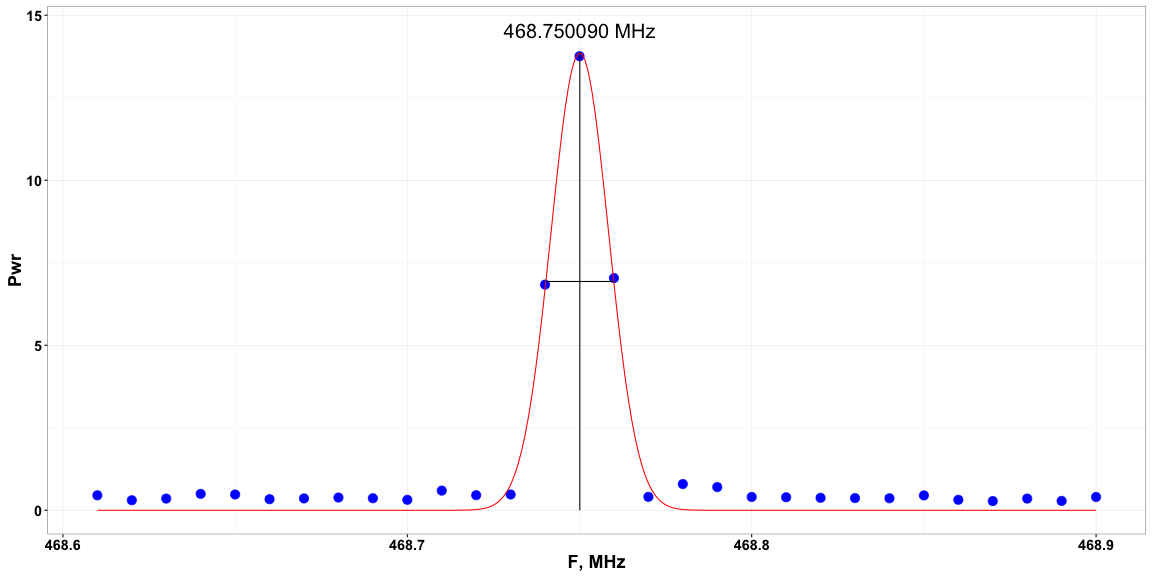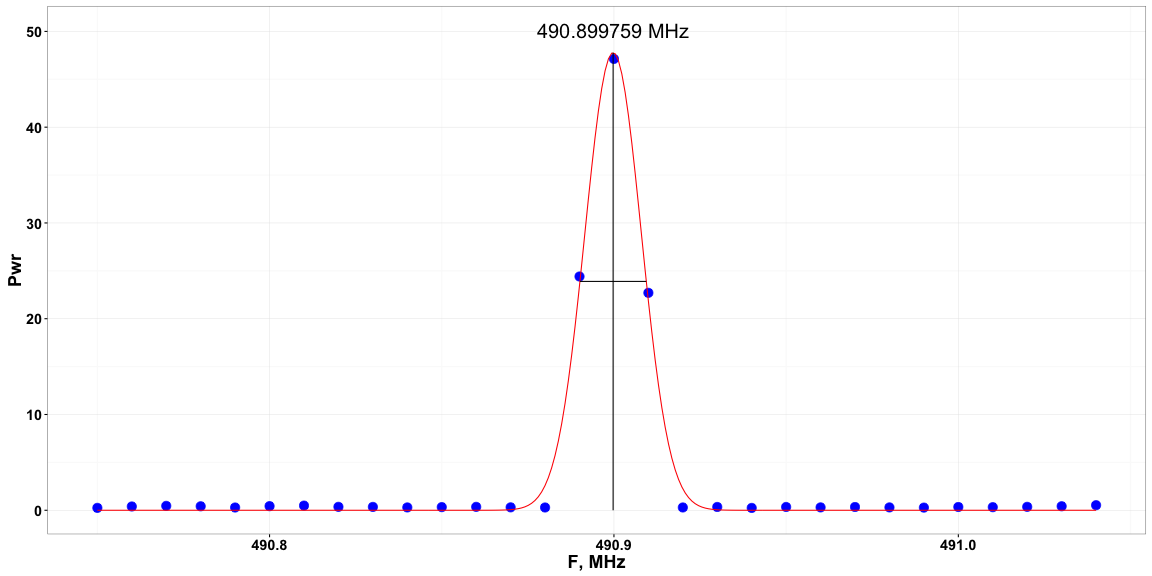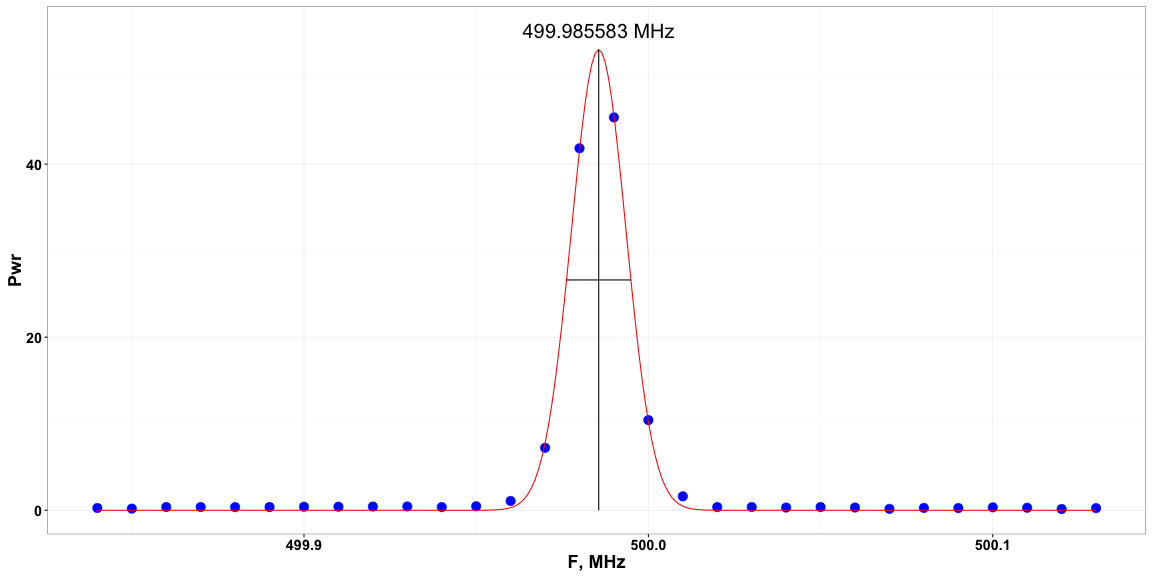Each fitted peak is shown with raw data, the fitted Gaussian, and lines representing the fitted centroid and FWHM (full width at half maximum). Note that this technique works only for a single isolated peak at a time. Overlapping peaks need to be fit together.

## Compare Measured and Actual Frequencies

``````## Compute numbers to be used to compare fitted and actual frequencies

channel_width_hz <- 1e6 * (display_data\$F - display_data\$F)

ActualFrequency <- c(0, 490.9, 499.985876) # Hardcoded settings from frequency synthesizers in MHz, if zero it's an unknown peak

Deltas <- NULL

for (i in 1:nrow(FitPeaks)) {
if (ActualFrequency[i] > 0) {
t.df <- data.frame(list(ActualF = 1e6 * ActualFrequency[i], # Numbers in Hz
FitF = 1e6 * FitPeaks\$Centroid[i],
SEF = 1e6 * FitPeaks\$CentroidSE[i],
ChannelWidth = channel_width_hz,
MaxChannel = 1e6 * FitPeaks\$InitialCentroid[i]
))
Deltas <- rbind(Deltas, t.df)
}
}

Deltas <- Deltas %>% mutate(PctDiff = 100 * (ActualF - FitF) / ActualF,
dF = ActualF - FitF, dFnofit = ActualF - MaxChannel,
ActualFstring = sprintf('%0.6f MHz', ActualF / 1e6))

Deltas # List the computed data
``````
``````##     ActualF      FitF      SEF ChannelWidth MaxChannel       PctDiff
## 1 490900000 490899759 247.1251        10000  490900000 0.00004911137
## 2 499985876 499985583 107.7334        10000  499990000 0.00005860380
##         dF         dFnofit  ActualFstring
## 1 241.0877     0.001075983 490.900000 MHz
## 2 293.0107 -4123.998903990 499.985876 MHz
``````
``````PointSize <- 5

for (i in 1:nrow(Deltas)) {
PlotDF <- NULL

PlotDF <-                data.frame(list(Item = 'Channel Width', x = Deltas\$MaxChannel[i] - (channel_width_hz / 2) ))
PlotDF <- rbind(PlotDF , data.frame(list(Item = 'Channel Width', x = Deltas\$MaxChannel[i]) ))
PlotDF <- rbind(PlotDF , data.frame(list(Item = 'Channel Width', x = Deltas\$MaxChannel[i] + (channel_width_hz / 2) )))

PlotDF <- rbind(PlotDF , data.frame(list(Item = 'Fit Frequency', x =  Deltas\$FitF[i] - Deltas\$SEF[i]) ))
PlotDF <- rbind(PlotDF , data.frame(list(Item = 'Fit Frequency', x =  Deltas\$FitF[i] ) ))
PlotDF <- rbind(PlotDF , data.frame(list(Item = 'Fit Frequency', x =  Deltas\$FitF[i] + Deltas\$SEF[i]) ))

PlotDF <- rbind(PlotDF , data.frame(list(Item = 'Acutal Frequency', x = Deltas\$ActualF[i]) ))

Title <- paste('100 * (ActualF - MeasuredF) / MeasuredF = ', formatC(Deltas\$PctDiff[i], format='e', digits = 3), '%')

p <- ggplot(PlotDF) + geom_line(aes(x = x / 1e6, y = Item), color = 'blue', size = PointSize / 5) +
geom_point(aes(x = x / 1e6, y = Item), color = 'blue', size = PointSize) +
xlab('F, MHz') + ylab('') + ggtitle(Title) + theme_jm2

print(p)
}
``````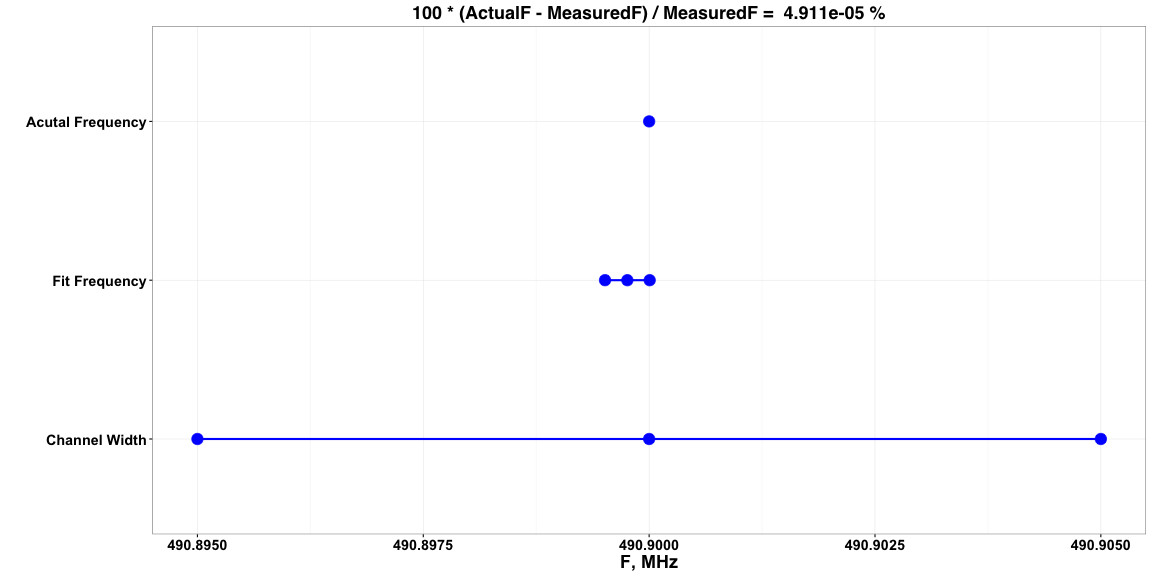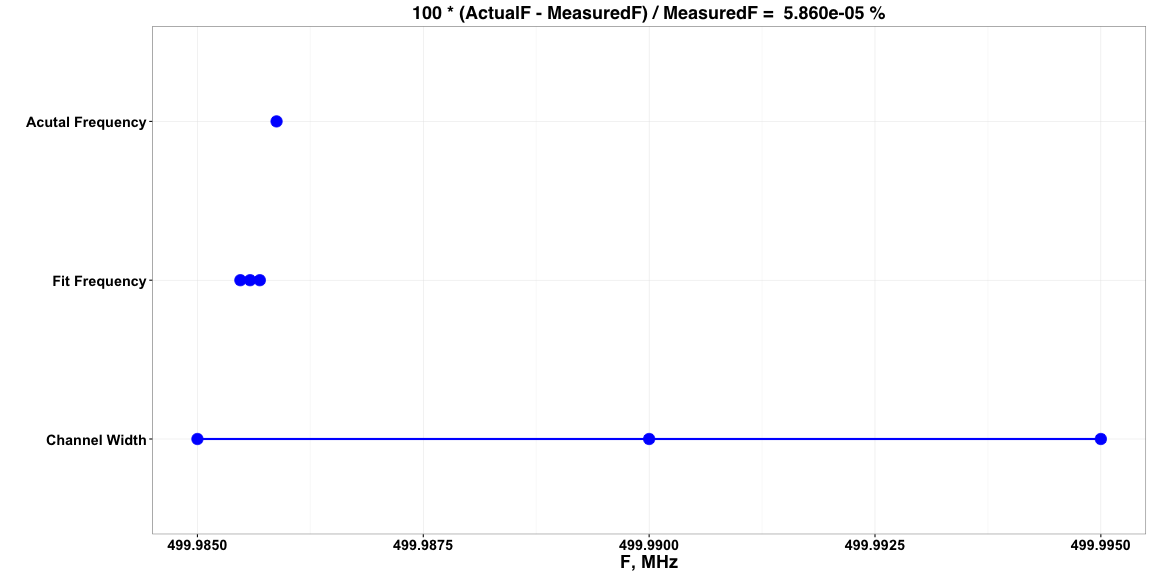An attempt to visualize the value of peak fitting for frequency measurements for the two frequency synthesizer peaks. “Channel Width” shows the channel with the maximum signal and the total channel width in MHz, one half of the spacing between channels is shown on each side of the maximum channel. “Fit Frequency” shows the fitted frequency in the center and the standard error of the fit on each side. Finally “Actual Frequency” shows the frequency to which the frequency synthesizers were set.

The 490.9 MHz peak happens to fall directly on a channel of the frequency spectrum and the fit slightly skews the measurement. However, the 499.985876 MHz peak falls close to halfway between channels and the peak fit improves the result significantly.

Finally, let's look at the percent error if the actual frequency fell exactly between two channels and we used the maximum height channel as the measurement. At 500 MHz this would be:

``````half_channel_width_Mhz <- channel_width_hz / 2e6
NoFitError <- 100 *  half_channel_width_Mhz / 500
``````

1.000e-03 %, a factor of about 17 better than just using the channel with maximum height for the 499.985876 MHz peak.

In the future we will extend this technique to multiple overlapping peaks and add a background noise level term.

\$Id: peak_fit_500MHz.Rmd,v 1.4 2016/11/28 23:22:15 meekj Exp \$